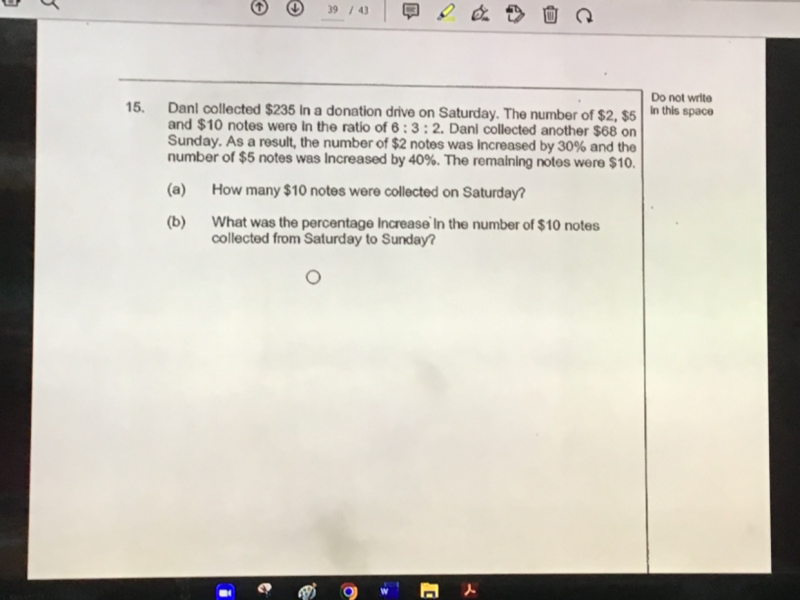# QuestionPls solve (b), thanks

\$2                \$5               \$10

Sat         6u                 3u                2u                = 235

Sun      1.3(6u)      1.4(3u)              ?                 = 235 + 68 = 303

(a)    6u(2 + (3u)5 + (2u)10 = 235

12u + 15u + 20u = 235

47u = 235 => u =5

Therefore, 2u = 10   \$10 notes collected on Saturday.

(b) 6u = 6×5 = 30,    3u = 3×5 =15

Let p be the number of \$10 notes on Sunday

(1.3)(30)(2) + 1.4(15)(5) + p(10) = 303

78 + 105 + 10 p = 303 => p = 120/10 = 12

Therefore, percentage of increase in \$10 from Sat to Sun =  (12-10)/10 x 100% = 20%